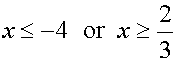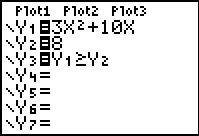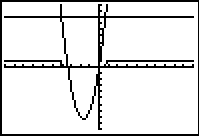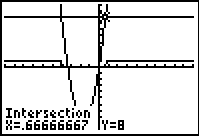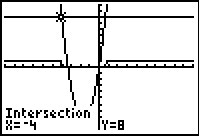Working with quadratic inequalities in one variable is similar to working with linear inequalities in one variable.  (See Linear Inequalities)

Consider the following examples:

 1. Solve: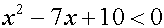1.  Enter the left side of the inequality into Y1. 2.  Enter the right side of the inequality into Y2. 3.  Enter the inequality into Y3 using the inequality symbols found under 2nd MATH (TEST).  Remember the Y1 and Y2 are found under Vars →Y-VARS, #1Function.  4.  Hit GRAPH. Just as we saw in Linear Inequalities, a small bar appears representing the graph of the value 1, showing where the inequality is true.  In this case, the inequality is true for  2 < x < 5.  To determine the "exact" values for the endpoints, use the INTERSECT option (2nd TRACE (CALC) #5 intersect) with Y1 and Y2. Looking at the table shows that the Y3 values alternate between being a 0 (false) and a 1 (true). (Arrow to the right to "see" the column for Y3. Remember that < will not "include" the endpoints.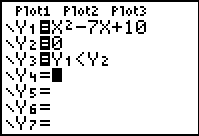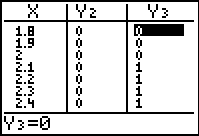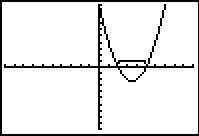2. Solve: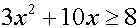1.  Enter the left side of the inequality into Y1. 2.  Enter the right side of the inequality into Y2. 3.  Enter the inequality into Y3 using the inequality symbols found under 2nd MATH (TEST).  Remember the Y1 and Y2 are found under Vars →Y-VARS, #1Function.  4.  Hit GRAPH.   The small bars appear representing the graph of the value 1, showing where the inequality is true.     Use the INTERSECT option (2nd TRACE (CALC) #5 intersect) with Y1 and Y2 to determine the exact values of the endpoints of the bars.  This inequality is true for